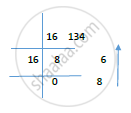# Convert 0010 0100 0010 1101 from Base 2 to Decimal. Convert 134 from Base 10 to Hexadecimal. Write Steps for Conversion. - Structured Programming Approach

Convert 0010 0100 0010 1101 from base 2 to decimal. Convert 134 from base 10 to hexadecimal. Write steps for conversion.

#### Solution

Binary to decimal: The steps to be followed are:

1. Multiply the binary digits (bits) with powers of 2 according to their positionalweight.
2. The position of the first bit (going from right to left) is 0 and it keeps on incrementing as you go towards left for every bit.
3.Given: (0010 0100 0010 1101)2 = (?)10
4.0*215+0*214+1*213+0*212+0*211+1*210+0*29+0*28+0*27+0*26+1*25+0*24+1*23+1*22+0*21+1*20
=0+0+8192+0+0+1024+0+0+0+0+32+0+8+4+1
=9261
(0010 0100 0010 1101)2 = (9261)10

Decimal to hexadecimal: The steps to be followed are:
1.Divide the decimal number by 16. Treat the division as an integer division.
2.Write down the remainder (in hexadecimal).
3.Divide the result again by 16. Treat the division as an integer division.
4.Repeat step 2 and 3 until result is 0.
5.The hexadecimal value is digit sequence of the reminders from last to first. 6.Given: (134)10 = (?)16(134)10 = (86)16

Concept: Turing Model
Is there an error in this question or solution?
2016-2017 (June) CBCGS

Share